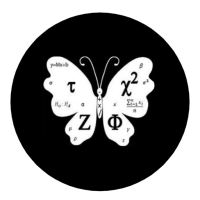## Welcome to Moore Statistics Consulting LLCYour teacher asks you to generate a correlation between three variables: dstat (disease),  wt (weight), and age (age). So you use SAS  or SPSS statistical software and come up with the following output:

 wt age dt wt 1 -0.3 0.43 age -0.3 1 0.5 dt 0.43 0.5 1

The numbers in this matrix represent your correlation values (r). How in the world do you interpret this matrix? The rows symbolize the correlations for a particular variable. The columns represent the second variable being compared to the row. So for instance, the correlation between age and wt is -0.3. The correlation between dt and age is 0.5. Why is the correlation between a variable  and itself ‘1’? Because the relationship between a variable and itself should bee 100% in agreement or 1. Why are we getting repeats of the numbers ? Because the comparison is in a matrix format. (Don’t look too deep if this is an introductory statistics course).

-Moore to follow- Amy